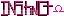-=+=- -=+=- -=+=- -=+=- -=+=- -=+=- -=+=- -=+=- -=+=- -=+=- -=+=- -=+=- -=+=- -=+=- -=+=- -=+=- -=+=- -=+=- -=+=- -=+=- -=+=- -=+=- -=+=- -=+=- -=+=- -=+=- -=+=- -=+=- -=+=- -=+=- (c) WidthPadding Industries 1987 0|150|0 -=+=- -=+=- -=+=- -=+=- -=+=- -=+=- -=+=- -=+=- -=+=- -=+=- -=+=- -=+=- -=+=- -=+=- -=+=- -=+=- -=+=- -=+=- -=+=- -=+=- -=+=- -=+=- -=+=- -=+=- -=+=- -=+=- -=+=- -=+=- -=+=- -=+=-
SoCoder -> Article Home -> Advanced Techniques

SchererererCreated : 31 January 2009
Edited : 31 January 2009

### Logic (part 2)

Part of the Series on Discrete Structures

Sunday, 01 February 2009, 13:37
PhoenixGood article, although slightly concise. What is the point of all those different rules of logic?

Tip: when you have those fancy characters, they are translated to something along the lines of "&xxxx;" in the HTML. This leaves a naughty semi-colon at the end, which in addition to a parenthesis gives you a misplaced smiley. So instead of typing (&xxxx;), type (&xxxx;[b][/b]).
Monday, 02 February 2009, 07:46
Schererererhmm... sounds like a glitch in jay's code! I'm not typing the unicode in directly, I'm just copying the character out of the character map in windows... I'll pm jay.

I know this article is a bit concise, but I'll get along to what those rules are used for soon. I probably should have waited to post this one until after I finished the other part of it, but oh well. The second part may come in as an edit of this article, I'll look at flow and then set it up however it all comes together nicest.Monday, 02 February 2009, 08:01
JayenkaiIt's to do with the way around that the data's being processed.. To keep it all safe, I do the "Unicode"-->&xxxx; thing early on. Otherwise, if you try sticking Unicode through php, there's all kinds of security holes that turn up, and all sorts.. Then you've the smiley code that comes after it..
You needn't have [b][/b], though.. a simple space will suffice!

Now, to be honest, I read, and re-read the cookie example, and although I understand it, it still doesn't make any sense in my head!
It's like the "Type" example in Blitz, with the chairs.. Although, technically, it makes sense, it really means bog all in my head!
Like most thing, give this a coded purpose, and my head will probably wrap around the logic a whole lot better.
I seem to be rubbish at learning from explainations!!Monday, 02 February 2009, 12:52
SchererererUnfortunately the idea is that a lot of this isn't code, it's the theory behind the code. <@Phoenix> this is a lot of the reason I wanted a separate article, because implication is such a funny topic at first </@>. But, I suppose if you were to use a code example:

Okay, in this example, we're testing a variable num to see if it is greater than or equal to 6. We'll take this as being equivalent to p(8594)q, where p is the "if (num >= 6)" and q is the "printf("Hello, World!");". If p, then q;.

So, if p is true (p=1), then in order for the statement p implies q (p(8594)q) to be true, q must be true (must execute). This means that, the only way for p(8594)q to be false, is if "Hello, World!" is printed without num being greater than or equal to 6.

Now, in the scenario where p=0 (num<6), it doesn't matter whether q is true or not ("Hello, World!" is printed or not). Therefore, for all values of q, where p=0, p(8594)q always evaluates to be true.

With a computer, basically think of implies as "does the algorithm work or not".